RD Sharma Test: Polynomials

# RD Sharma Test: Polynomials

Test Description

## 25 Questions MCQ Test Mathematics (Maths) Class 10 | RD Sharma Test: Polynomials

RD Sharma Test: Polynomials for Class 10 2022 is part of Mathematics (Maths) Class 10 preparation. The RD Sharma Test: Polynomials questions and answers have been prepared according to the Class 10 exam syllabus.The RD Sharma Test: Polynomials MCQs are made for Class 10 2022 Exam. Find important definitions, questions, notes, meanings, examples, exercises, MCQs and online tests for RD Sharma Test: Polynomials below.
Solutions of RD Sharma Test: Polynomials questions in English are available as part of our Mathematics (Maths) Class 10 for Class 10 & RD Sharma Test: Polynomials solutions in Hindi for Mathematics (Maths) Class 10 course. Download more important topics, notes, lectures and mock test series for Class 10 Exam by signing up for free. Attempt RD Sharma Test: Polynomials | 25 questions in 25 minutes | Mock test for Class 10 preparation | Free important questions MCQ to study Mathematics (Maths) Class 10 for Class 10 Exam | Download free PDF with solutions
 1 Crore+ students have signed up on EduRev. Have you?
RD Sharma Test: Polynomials - Question 1

### If the graph of a polynomial intersects the x – axis at three points, then the number of zeroes =

Detailed Solution for RD Sharma Test: Polynomials - Question 1

If the graph of a polynomial intersects the x-axis at three points, then the number of zeroes are 3 because number of zeroes of the polynomial are the number of the coordinates of the points where its graph intersects the x-axis.

RD Sharma Test: Polynomials - Question 2

### If ‘α’ and ‘β’ are the zeroes of a quadratic polynomial x2+ 5x − 5, then

Detailed Solution for RD Sharma Test: Polynomials - Question 2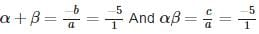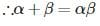RD Sharma Test: Polynomials - Question 3

### The sum and product of the zeroes of the polynomial x2−6x+8 are respectively

Detailed Solution for RD Sharma Test: Polynomials - Question 3

Sum of the zeroes of the polynomial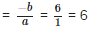And Product of the zeroes of the polynomial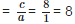RD Sharma Test: Polynomials - Question 4

The number of zeros of a cubic polynomial is

Detailed Solution for RD Sharma Test: Polynomials - Question 4

A polynomial of degree 3 is called a cubic polynomial.

For example, x3−1, 4a− 100a+ a − 6, and m2n + mn2 are all cubic polynomials with atmost 3 zeroes (having degree 3).

Hence, the polynomial having atmost 3 zero is a cubic polynomial.

RD Sharma Test: Polynomials - Question 5

If x3+x2−2x−3 = (x−2)(x2+ax+b)+5, then

Detailed Solution for RD Sharma Test: Polynomials - Question 5

Given: x3+x2−2x−3 = (x−2)(x2+ax+b)+5

Dividing L.H.S. by (x−2)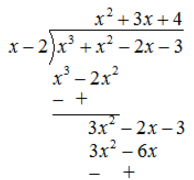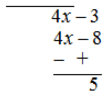∴ (x-2)(x2+3x+4)+5 = (x-2)(x2+az+b)+5
Comparing both side, we have a = 3, b = 4

RD Sharma Test: Polynomials - Question 6

The graph of the polynomial f(x) = 2x – 5 intersects the x – axis at

Detailed Solution for RD Sharma Test: Polynomials - Question 6

If The graph of the polynomial f(x) = 2x – 5 intersects the x – axis then y = 0
2x−5 = 0 ⇒ x = 5/2

RD Sharma Test: Polynomials - Question 7

If ‘α ’ and ‘β ’ are the zeroes of a quadratic polynomial x2− 5x + b and α − β = 1, then the value of ‘b’ is

Detailed Solution for RD Sharma Test: Polynomials - Question 7

Here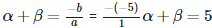……….(i)
And it is given that α−β = 1 ……….(ii)
On solving eq. (i) and eq. (ii),
we get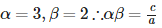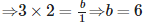RD Sharma Test: Polynomials - Question 8

If one of the zeroes of the cubic polynomial x3−7x+6 is 2, then the product of the other two zeroes is

Detailed Solution for RD Sharma Test: Polynomials - Question 8

Let α,β,γ are the zeroes of the given polynomial. Given: α = 2
Since αβγ = -d/a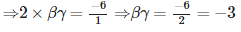RD Sharma Test: Polynomials - Question 9

The maximum number of zeroes that a polynomial of degree 3 can have is

Detailed Solution for RD Sharma Test: Polynomials - Question 9

The maximum number of zeroes that a polynomial of degree 3 can have is three because the number of zeroes of a polynomical is equals to the degree of that polynomial.

RD Sharma Test: Polynomials - Question 10

The real number which should be subtracted from the polynomial 2x3+5x2−14x+10 so that the polynomial 2x−3 divides it exactly is

Detailed Solution for RD Sharma Test: Polynomials - Question 10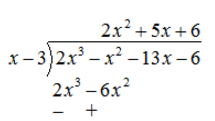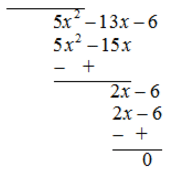Therefore, 7 should be subtracted.

RD Sharma Test: Polynomials - Question 11

The graph of a cubic polynomial x3 – 4x meets the x – axis at (– 2, 0), (0, 0) and (2, 0), then the zeroes of the polynomial are

Detailed Solution for RD Sharma Test: Polynomials - Question 11

If the graph of a cubic polynomial intersects thrice x-axis, then the zeroes of the cubic polynomial are coordinates of x-axis.

RD Sharma Test: Polynomials - Question 12

If one zero of the quadratic polynomial x2+ 3x + k is 2, then the value of ‘k’ is

Detailed Solution for RD Sharma Test: Polynomials - Question 12

According to question, p(2) = 0
⇒ (2)2+3×2+k = 0
⇒ 4+6+k = 0
⇒ k = −10

RD Sharma Test: Polynomials - Question 13

If the sum of the zeroes of the cubic polynomial 4x3−kx2−8x−12 is -3/4  then the value of ‘k’ is

Detailed Solution for RD Sharma Test: Polynomials - Question 13

Let α,β,γ are the zeroes of the given polynomial. Given: α+β+γ = (-3/4)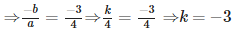RD Sharma Test: Polynomials - Question 14

If α and β are the zeroes of the polynomial 2x2+5x+1, then the value of α+β+αβ is

Detailed Solution for RD Sharma Test: Polynomials - Question 14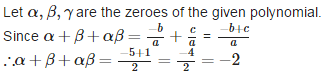RD Sharma Test: Polynomials - Question 15

If √2 and −√2 are the zeroes of 2x4−3x3−3x2+6x−2, then the other zeroes are

Detailed Solution for RD Sharma Test: Polynomials - Question 15

Since √2 and −√2 are the zeroes of 2x4−3x3−3x2+6x−2, then(x−√2) (x+√2) are the factors of given polynomial i.e.,
(x−√2) (x+√2) = (x2−2) is a factor of given polynomial.
∴p(x) = 2x4−3x3−3x2+6x−2
⇒ p(x) = (x2−2)(2x2−3x+1)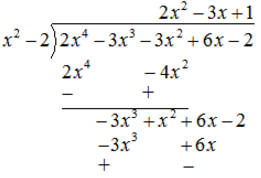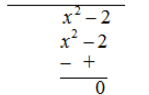⇒ p(x) = (x2 - 2) [2x2 - 2x - x + 1]
⇒ p (x) = (x2 - 2) [2x (x -1) - 1 (x - 1)]
⇒ p (x) = (x2 - 2) (x -1) (2x -1)
∴ Other zeroes are x - 1 = 0 and 2x - 1 = 0 ⇒ x = 1 and x = 1/2

RD Sharma Test: Polynomials - Question 16

If one zero of the polynomial p(x) = (k+4)x2+13x+3k is reciprocal of the other, then the value of ‘k’ is

Detailed Solution for RD Sharma Test: Polynomials - Question 16

Let one zero of the given polynomial be athen the other zero be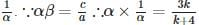⇒ k + 4 = 3k ⇒ 2k = 4 ⇒ k = 2

RD Sharma Test: Polynomials - Question 17

If two of the zeroes of a cubic polynomial ax3+bx2+cx+d are zero, then the third zero is

Detailed Solution for RD Sharma Test: Polynomials - Question 17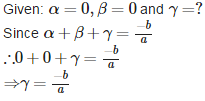RD Sharma Test: Polynomials - Question 18

If ‘2’ is the zero of both the polynomials 3x2+mx−14 and 2x3+nx2+x−2, then the value of m – 2n is

Detailed Solution for RD Sharma Test: Polynomials - Question 18

According to the question, p (2) = 3x2 + mx - 14 = 0
⇒ 3(2)2 + m x 2 - 14 = 0
⇒ 12 + 2m - 14 = 0 ⇒ m = 1
Also p(2) = 2x+ nx2 + x - 2 = 0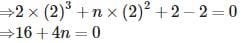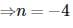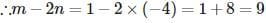RD Sharma Test: Polynomials - Question 19

If one zero of the polynomial p(x) = (a2+9)x2+45x+6a is reciprocal of the other, then the value of ‘a’ is

Detailed Solution for RD Sharma Test: Polynomials - Question 19

Let the zeroes be k and (1/k)
Given quadratic polynomial is p(x) = (a2 + 9)x2 + 45x + 6a
Recall the sum of zeroes (k + 1/k) = –45/(a2 + 9)
Product of zeroes [k × (1/k)] = 6a/(a2 + 9)
⇒ 6a/(a2 + 9) = 1
⇒ (a2 + 9) = 6a
⇒ a2 – 6a + 9 = 0
⇒ a2 – 2(a)(3) + 32 = 0
⇒ (a – 3)2 = 0
⇒ (a – 3) = 0
∴ a = 3

RD Sharma Test: Polynomials - Question 20

The polynomial to be added to the polynomial x4+2x3−2x2+x−1 so that the resulting polynomial is exactly divisible by x2+2x−3 is

Detailed Solution for RD Sharma Test: Polynomials - Question 20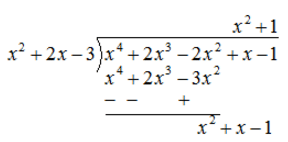Now (x2+2x−3)−(x2+x−1) = x−2
Therefore, (x−2) is the polynomial which to be added to the given polynomial.

RD Sharma Test: Polynomials - Question 21

The sum of two zeroes of the polynomial f(x) = 2x+ (p+3)x + 5 is zero, then the value of ‘p’ is

Detailed Solution for RD Sharma Test: Polynomials - Question 21

Let one zeroes of the given polynomial be α and β. According to the question,

Sum of the zeroes = -b/a = 0

⇒ -(p-3)/2 = 0
⇒ - (p-3) = 0×2

⇒ - (p-3) = 0

⇒ p = - 3

RD Sharma Test: Polynomials - Question 22

A quadratic polynomial whose zeroes are - 3 and 6, is

Detailed Solution for RD Sharma Test: Polynomials - Question 22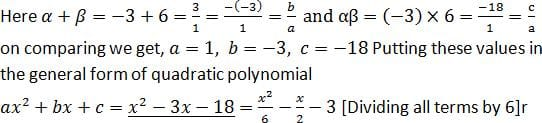RD Sharma Test: Polynomials - Question 23

A quadratic polynomial whose product and sum of zeroes are 1/3 and √2 respectively is

Detailed Solution for RD Sharma Test: Polynomials - Question 23

Given: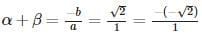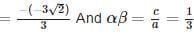On comparing, we get,a = 3,b = −3√2–,c = 1
Putting these values in general form of a quadratic polynomial ax2+bx+c, we have 3x2−3√2x+1

RD Sharma Test: Polynomials - Question 24

The sum and product of the zeroes of the polynomial f(x) = 4x2−27x+3k2 are equal, then the value of ‘k’ is

Detailed Solution for RD Sharma Test: Polynomials - Question 24

Let α,β are the zeroes of the given polynomial.
Given: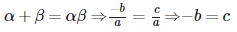⇒ −(−27) = 3k⇒ k2 = 0 ⇒ k= ±3

RD Sharma Test: Polynomials - Question 25

A polynomial of degree ____ is called a quadratic polynomial.

Detailed Solution for RD Sharma Test: Polynomials - Question 25

The term quadratic describes something that pertains to squares, to the operation of squaring, to terms of the second degree, or equations or formulas that involve such terms.It involves the second and no higher power of an unknown quantity or variable.

## Mathematics (Maths) Class 10

53 videos|369 docs|138 tests
 Use Code STAYHOME200 and get INR 200 additional OFF Use Coupon Code
Information about RD Sharma Test: Polynomials Page
In this test you can find the Exam questions for RD Sharma Test: Polynomials solved & explained in the simplest way possible. Besides giving Questions and answers for RD Sharma Test: Polynomials, EduRev gives you an ample number of Online tests for practice

## Mathematics (Maths) Class 10

53 videos|369 docs|138 tests